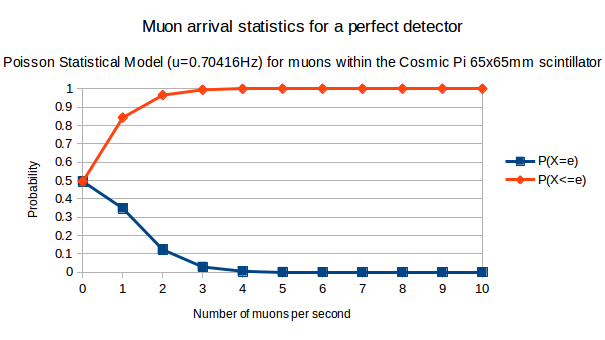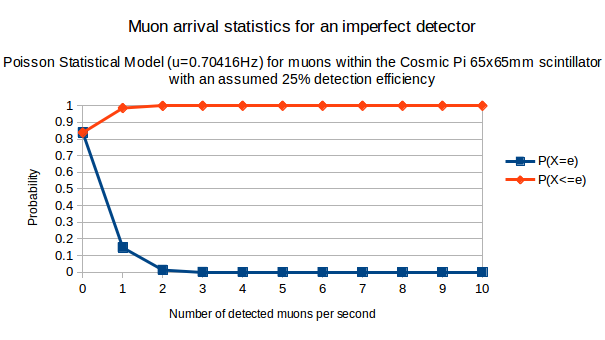One thing we've been trying to grapple with recently whilst setting up the detection parameters for our first batch of Cosmic Pi units is how many muons we should be detecting. In this post we'll be using the Poisson distribution to calculate the probability of a given muon arrival rate. Poisson is a fairly simple distribution and we only need one parameter (the expected rate) to make our calculations.

P(x; μ) = (e) (μx) / x!

x = the number of events

μ = the mean event rate

e = 2.71828...

As a starting point, let's take the generally accepted figure of merit for the arrival of muons at sea level of 1 muon per minute per square centimeter. We'll convert this into a rate per mm2 per second:

 0.000166667 Hz/mm^-2

And then apply this rate to the area (note, not the volume - we assume for this exercise that all muons have vertical trajectories) of the 65mm x 65mm Cosmic Pi scintillator, which has an area of  4225mm2:

 0.704167 Hz

Which gives us the expected mean rate for muons that will be passing through the slab in any given second. Since this is just an average value, there is also a probability that we will have 0 muons in a given second or more than 1 muon. This is very interesting to know, as it will help us to calibrate both the performance of the detector and our own expectations of what we should be detecting!

If the Cosmic Pi was a perfect muon detector, we would expect to see 100% of all muons passing through, which would give us the following statistical distribution:

 Events per second 0 1 2 3 4 5 6 7 8 9 10 P(X=e) 0.49 0.35 0.12 0.03 0.01 0 0 0 0 0 0 P(X<=e) 0.49 0.84 0.97 0.99 1 1 1 1 1 1 1

Note that P(X=e) is the probability of getting exactly e events (0, 1, 2 etc.) within a given second and P(X<=e) is the cumulative probability of getting less than or equal to e events within a given second.If the Cosmic Pi detected 100% of all muons passing through the scintillator, this is the statistical distribution we could expect.

A quick glance at the graph indicates that most seconds will have either 0 or 1 events. A smaller number will have 2 events and only 3% will have up to 3 events. This is good news, in that it means we can be relatively relaxed with regards to the electronics design, as an event rate exceeding 5 Hz is very unlikely. This isn't the case for LHC experiments, which experience a huge event rate and consequently suffer from pile up problems that need to be solved. We had calculated our expected maximum event rate before starting the design - which is why we used 5 Hz as the maximum.

However, the construction of a perfect detector is a virtually impossible task. Due to the limitations of the technology used (and the budget), Cosmic Pi isn't going to be able to detect all the muons that go through the slab. We are still working on quantifying the detection efficiency of the system, but for now let's assume that it's 25% (i.e. 1 in 4 of the muons we see is detected as an event, the other 3 escape). This has a significant impact on the statistical distribution we can expect:

 Events per second 0 1 2 3 4 5 6 7 8 9 10 P(X=e) 0.84 0.15 0.01 0 0 0 0 0 0 0 0 P(X<=e) 0.84 0.99 1 1 1 1 1 1 1 1 1

As you can see from the table and the graph below, the probability that we will now see 2 muons in a given second becomes very small at 1%.If we assume that only 25% of muons passing through a Cosmic Pi are detected, this is what we can expect.

In summary, we can therefore realistically expect that in a typical second 0 muons will be detected, with a 15% probability that one muon will be detected. This helps us to understand what kind of data we should be getting out of the unit and at a human level, how often the event indicator should flash if we're really seeing muons.